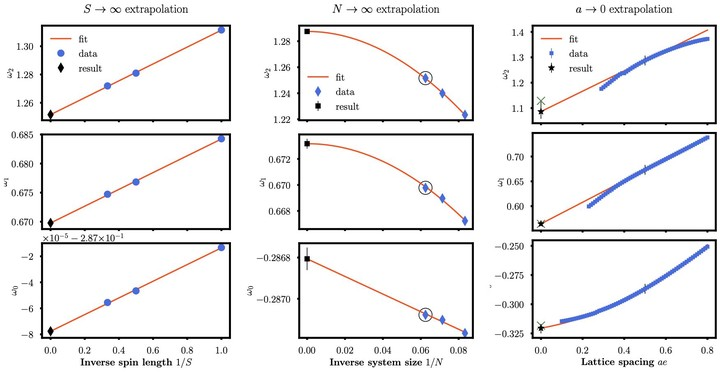# Towards the continuum limit of a $(1+1)$d quantum link Schwinger model### Abstract

The solution of gauge theories is one of the most promising applications of quantum technologies. Here, we discuss the approach to the continuum limit for $U(1)$ gauge theories regularized via finite-dimensional Hilbert spaces of quantum spin-$S$ operators, known as quantum link models. For quantum electrodynamics (QED) in one spatial dimension, we numerically demonstrate the continuum limit by extrapolating the ground state energy, the scalar, and the vector meson masses to large spin lengths $S$, large volume $N$, and vanishing lattice spacing $a$. By analytically solving Gauss’ law for arbitrary $S$, we obtain a generalized PXP spin model and count the physical Hilbert space dimension analytically. This allows us to quantify the required resources for reliable extrapolations to the continuum limit on quantum devices. We use a functional integral approach to relate the model with large values of half-integer spins to the physics at topological angle $\Theta=\pi$. Our findings indicate that quantum devices will in the foreseeable future be able to quantitatively probe the QED regime with quantum link models.

Type
Publication
Physical Review D 106, L091502
Published 3 November 2022